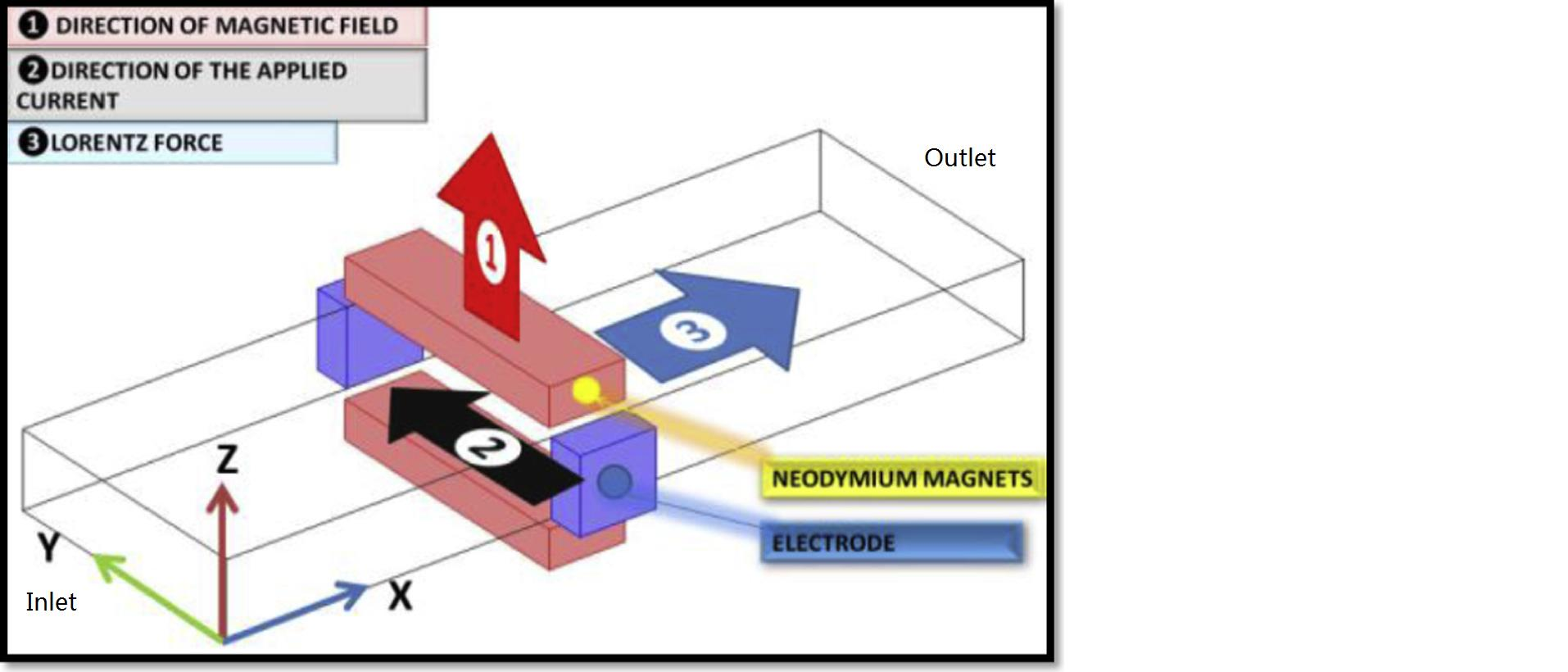# Which approach should I use when doing MHD simulation: magnetic induction or electric potential?

Hello! I want to use Fluent's MHD add-on module to simulate a liquid metal flow in an electromagnetic pump. Bases on the following post, it says that "Lorentz force effect on the fluid flow is accounted for only when the magnetic induction method is used. It is ignored in the electric potential method which applies Lorentz force only to DPM particles."  Is that correct? I don't see similar description in the Fluent MHD manual. If anyone can help me clarify it, I would appreciate it. Thanks.

• edited March 2019

Yes, this is correct in a sense that if an external magnetic field is not specified, then the electric potential implementation in the MHD module won't apply the Lorentz force acting on the fluid. While the manual doesn't explicitly state this, eq, (2-25) in Sec. 2.3. "Electric Potential Method" shows the Lorentz force acting on the fluid includes the magnetic field B, but not E. The electric potential method only calculates the electric field, E, thus the Lorentz force will be zero unless an external magnetic field in supplied. On the other hand, for charged DPM particles, Lorentz force calculation includes E, as shown in eq. (2-27).

• edited March 2019

Hello Kkourbat,

Thank you very much for your detailed answers. Since in my case, I apply an external magnetic field along with a electric potential, I assumed the Lorentz force will be calculated.

I have another question. It is an electromagnetic pump I am simulating, the pumping of the liquid metal will be caused by Lorentz force, but I don't know what inlet boundary condition would be appropriate. Velocity inlet with zero velocity or pressure inlet with zero total pressure?

Thanks,

• edited March 2019

If I understand your description correctly, the flow will be induced by the Lorentz force through the inlet into the domain. In this case I would recommend either pressure inlet with zero total pressure or pressure outlet with zero static pressure. In the latter case, pressure outlet will reverse and act as an inlet.

• edited March 2019

I will try that. Thank you.

• edited March 2019

Hello,

I am trying to model an electromagnetic pump. The model setup is like the following figure (just a rectangular channel). The fluid material is liquid lithium. I used electric potential MHD method in Fluent. The external magnetic field B0 is 0.7T at Z direction. The inlet is zero total pressure, outlet is zero static pressure. The left side wall has an electric potential of 0V, the right wall has an electric potential of 0.08V. The top and bottom walls are insulated.

I tried to use these setup to run the simulation, but I just can't get a converged result, the intermediate result looks strange. I was wondering if my boundary conditions are correct. If someone can give me some guidelines. I will appreciate it. Thanks,• edited March 2019

My suggestions are:

1) solve for the electric potential only by turning off flow equations, and verify if computed potential, current and Lorentz force are what you expect

2) solve for the flow without MHD as a sanity check

3) Lithium is quite conductive, and Lorentz force sources in the momentum equations will be large, which can impact convergence. You can try body force weighted scheme for pressure, P-V coupled, under-relax pressure and momentum to 0.2, and use Flow Courant Number of 20

• Hello All,

I simulated the Electromagnetic pump but the problem is that I can not calculate magnetic induction and the software assume that the amount of magnetic induction is zero. Is there any way to couple the MHD module (magnetic induction) via the Electrical potential method? when I use both, the software can not couple them just solve separately.

Thank you.

• edited February 3

Hello, it's not clear what you would like to couple and why. Tee MHD magnetic induction method should give you 1) magnetic field, 2) current, 3) Joule heating, 4) Lorentz force. Why do you need the electric potential?

• Hello,

It would be also of my interest to learn if you can output Electric Potential when using the Magnetic Induction Method. Consider for example MHD flow in a rectangular duct (in relation to nuclear fusion reactor cooling blankets). It has external magnetic field and induced currents resulting in Electric Potential difference between side walls. Electric Potential difference happens to be one of the few things that can be measured experimentally to assess the flow pattern. For such a simulation it would be necessary to use the Magnetic Induction Method and be able to output Electric Potential data at the end.

So the question is: How to get Electric Potential results when using the Magnetic Induction method in Fluent?

Thank you.